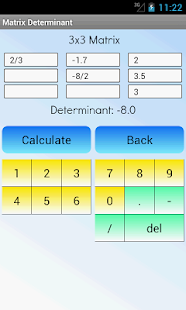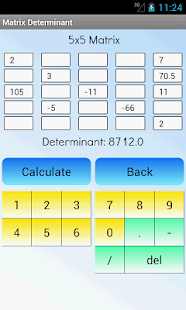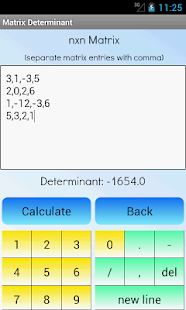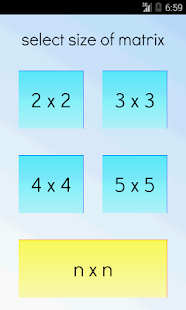Calculate the determinant of the matrix! Simple and fast!

This app is the pro version of the “matrix determinant calculator”, completely ad-free!

This app is a math calculator, which is able to calculate the determinant of a matrix. The determinants of the following matrices are available:

– 2×2 arrays
– 3×3 arrays
– 4×4 arrays
– 5×5 arrays
– nxn arrays (with more than 5 rows and columns)

Best math tool for school and college! If you are a student, it will help you learn linear algebra!

What’s New:
No changelog

••••